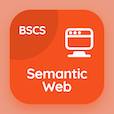Online CS Degree Courses

Digital Logic Design MCQs

Digital Logic Design MCQ - Topics

Learn Subtraction With Complement Multiple Choice Questions (MCQ Quiz), Subtraction With Complement quiz answers PDF to learn digital logic design course for digital logic design online classes. Binary Systems Multiple Choice Questions and Answers (MCQs), Subtraction With Complement quiz questions for online computer science and engineering. Subtraction With Complement Book PDF: binary code, subtraction with complement, definition of binary logic, logic gates in digital logic design test prep for online software development courses.

"Using 10's complement subtracting 3250 from 72532 is equal to" MCQ PDF: subtraction with complement App APK with 69282, 69272, 69252, and 69232 choices for online computer science and engineering. Study subtraction with complement quiz questions for merit scholarship test and certificate programs to learn free online courses.

## MCQs on Subtraction With Complement Quiz

MCQ: Using 10's complement subtracting 3250 from 72532 is equal to

69282
69272
69252
69232

MCQ: X=1010100 and Y=1000011 using 2's complement X-Y is

1011101
10110010
1001100
10001

MCQ: Using 10's complement, subtracting 72532 from 3250 is equal to

-69272
-69282
-69252
-69232

MCQ: X=1010100 and Y=1000011 using 1's complement Y-X is

-1011110
-1001001
-10001
-1100100

### More Quizzes on Digital Logic Design Book